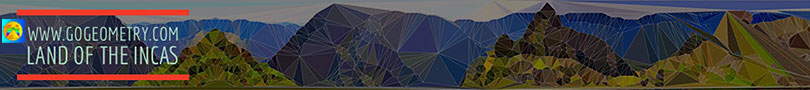# Geometry Problem 164. Parallelogram, Trapezoid, Diagonal, Triangles, Areas

 In the figure below, ABCD is a parallelogram with E any point on side BC and F the point of intersection of AE and BD. If S, S1, and S2 are the areas of triangles AFD, BEF, and CDE respectively, prove that S = S1 + S2. View or post a solutionHome | Geometry | Search | Problems | 161-170 | Area of a Parallelogram | Email | By Antonio Gutierrez